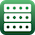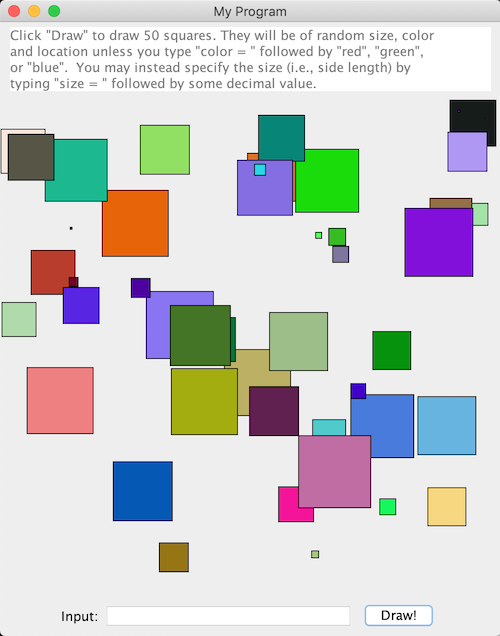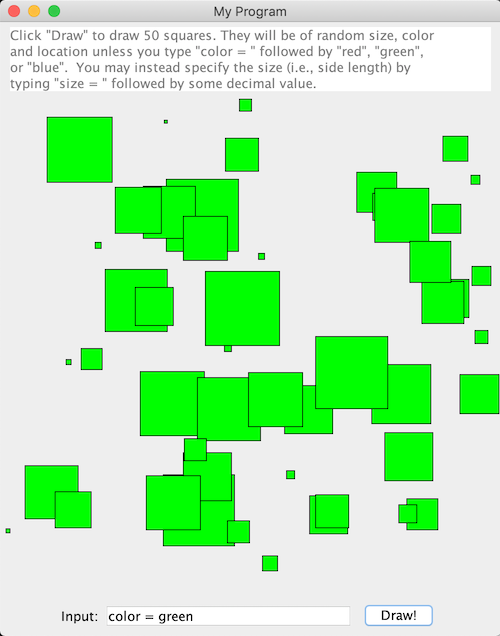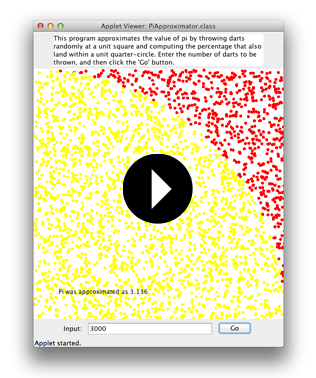Exercises - Loops

Some of the exercises below provide sample runs to better clarify what the program in question is supposed to do. In these sample runs, text given in green has been typed by the user, while white text has been output by the program. Additionally, the "$" symbol indicates the command prompt, while the "↵" symbol indicates the user has pressed the return key. Exercises identified with a breadboard logo(shown at left) requires the following library: breadboards.jar. 1. Write a class named ReverseNumber that takes a single positive integer as a command line argument and prints it out in reverse order.$ java ReverseNumber 192837↵
738291

2. Write a class named MeanFinder that prompts the user to type in some number of decimal values separated by spaces and then displays the mean value of the numbers entered.

$java MeanFinder↵ Enter some number of values, separated by spaces: 1 3 5.6 7.89↵ The mean of the values entered is 4.3725 3. Write a class called SumOfDigitsFinder whose main method prompts the user for an integer, and then finds and displays the sum of the digits of that integer. 4. An integer is called a palindrome if its digits can be reversed without changing its value. For example, 15351, and 7227 are both palindromes, while 12345 is not. Write a class called CheckIfPalindrome that takes a positive integer command line argument and indicates to the user whether or not this value is a palindrome. Sample runs follow:$ java CheckIfPalindrome 1234321↵
1234321 is a palindrome.

$java CheckIfPalindrome 1234↵ 1234 is not a palindrome. 5. Write a class named AdditionQuiz that prompts the user to answer randomly generated addition questions in such a way that: • Each time the program is run, different numbers should appear. • The numbers to be added should each be less than 100. • The questions should continue to be generated until the user gets 5 of them correct in a row. A sample run follows:$ java AdditionQuiz↵
This program will quiz you on simple addition.
Answer five in a row correctly to stop.
----------------------------------------------
49 + 70 = 119↵
correct!

44 + 67 = 112↵
whoops!

87 + 71 = 158↵
correct!

25 + 35 = 60↵
correct!

0 + 78 = 78↵
correct!

50 + 59 = 109↵
correct!

81 + 61 = 142↵
correct!

Good job! You got five in a row!

import java.util.Random;
import java.util.Scanner;

public static void main(String[] args) {
System.out.println("This program will quiz you on simple addition.\n"
+ "Answer five in a row correctly to stop.\n"
+ "----------------------------------------------");

// this will be needed later to produce random numbers..
Random random = new Random();

// this will be needed later to collect user input..
Scanner scanner = new Scanner(System.in);

int numCorrect = 0;

while (numCorrect < 5) {
// generate two random numbers to add, and find their sum..
int a = random.nextInt(100);
int b = random.nextInt(100);
int correctSum = a + b;

// display the problem just generated..
System.out.print( a + " + " + b + " = ");

int enteredValue = Integer.parseInt(scanner.nextLine());
if (enteredValue == correctSum) {
numCorrect++;
System.out.println("correct!\n");
}
else {
System.out.println("whoops!\n");
}
}

System.out.println("Good job! You got five in a row!");
}
}

6. Write a class named Gcd that takes two positive integers as command line arguments, and then displays the calculations done to determine the greatest common divisor of those integers. Amazingly, these calculations do not require factoring the initial integers! One can find the gcd of two integers a and b by finding the remainder r of their quotient, and then repeating this process letting a = b and b = r until the remainder is zero. The last non-zero remainder is the gcd. The sample run below demonstrates this process.

$java Gcd 54321 9876↵ 54321 = 5 * 9876 + 4941 9876 = 1 * 4941 + 4935 4941 = 1 * 4935 + 6 4935 = 822 * 6 + 3 6 = 2 * 3 + 0 gcd = 3 7. Write a class named BaseChange, that when executed takes two command line arguments: a base-10 positive integer and a positive integer base into which to convert the base-10 number, and then prints to the console the converted value. When the base is 36 or less, use capital letters to represent the digits past 9 in a manner similar to that used in hexadecimal notation. When the base is greater than 36, denote the digits by their numerical values (possibly exceeding 9), but separate these digits by spaces (as shown in the second sample run). When writing the code for this class, implement your own algorithm to perform the base conversion, rather than relying on any pre-made methods to this end.$ java BaseChange 1012 16↵
3F4

$java BaseChange 4529 39↵ 2 38 5 8. Write a class named PascalsTriangle that takes a positive integer$n$as a command line argument and then displays Pascal's triangle up to row$n$. The values in each row should be found using the method of successive multiplications described here. Sample run:$ java PascalsTriangle 10↵
1
1  1
1  2  1
1  3  3  1
1  4  6  4  1
1  5  10  10  5  1
1  6  15  20  15  6  1
1  7  21  35  35  21  7  1
1  8  28  56  70  56  28  8  1
1  9  36  84  126  126  84  36  9  1
1  10  45  120  210  252  210  120  45  10  1

Also write a class named EvenOddTriangle that does the exact same thing as the program above, but instead of printing the numerical values in each row of Pascal's triangle, print either an 'E' if the corresponding value was even and an 'O' if the value was odd, without any spaces in between. You may be surprised at what you see!

9. In the dice game of craps, one rolls two dice and then one of several things happen:

• If you roll a 7 or 11, you win immediately
• If you roll a 2, 3, or 12, you lose immediately
• If you roll any other number (called the "point"), you continue to roll the dice until you see either a 7 or the "point" again). If you see a 7 first, you lose. If you see the "point" first, you win.

Write a class named ConsoleCraps that simulates a game of craps in a manner consistent with the sample runs shown below:

$java ConsoleCraps↵ 1st Roll = 7 WIN!$ java ConsoleCraps↵
1st Roll = 3
LOSE!

10. $java ConsoleCraps↵ 1st Roll = 5 Point = 5 Roll = 6 Roll = 3 Roll = 11 Roll = 7 LOSE!$ java ConsoleCraps↵
1st Roll = 10
Point = 10
Roll = 4
Roll = 12
Roll = 2
Roll = 10
WIN!

11. A proper divisor of a positive integer $n$ is an integer $d$ that divides $n$ evenly (i.e., with a remainder of $0$) with $0 \lt d \lt n$. A number is said to be perfect if it equals the sum of its proper divisors. For example, $6 = 1 + 2 + 3$ and $28 = 1 + 2 + 4 + 7 + 14$, so both $6$ and $28$ are perfect numbers.

Alternatively, if the sum of a number's proper divisors exceeds the number itself, it is said to be abundant, while if the sum of a number's proper divisors is less than the number itself, it is said to be deficient.

1. Write a class named ClassifyNumbersBelow that takes a single positive integer $n$ as a command line argument and then classifies the positive integers from 2 to $n$ as either abundant, deficient, or perfect. Include in your code a method named sumProperDivisors() that calculates the sum of the proper divisors of a given number (so that, System.out.print(sumProperDivisors(12)); will print 16). A sample run follows:

$java ClassifyNumbersBelow 20↵ 2 is deficient 3 is deficient 4 is deficient 5 is deficient 6 is perfect 7 is deficient 8 is deficient 9 is deficient 10 is deficient 11 is deficient 12 is abundant 13 is deficient 14 is deficient 15 is deficient 16 is deficient 17 is deficient 18 is abundant 19 is deficient 20 is abundant 2. Perfect numbers are actually very rare. In fact, as of today, there are only 49 perfect numbers known to exist! The last one was just discovered just in 2016! (See the list.) Interestingly, all of the known perfect numbers happen to be even, but nobody has proven that an odd one can't exist --if you should find one, you'll be quite famous mathematically! Write a class named FindPerfectNumbersBelow that takes a single positive integer$n$as a command line argument, and then prints all perfect numbers from 2 to$n$. As you do this, re-use the method sumProperDivisors() mentioned in part (a) above. Also, as calculating these numbers can take some time, and the user might get confused as to whether the program has concluded or just in the middle of a lengthy computation -- the word done should be printed when the method concludes. Consider the following sample run: Find perfect numbers below what positive integer n? 500↵ 6 28 496 done 12. Write a class named FactorIntoPrimePowers that takes a single positive integer as a command-line argument and then displays the prime factorization of that integer. As the below sample runs below suggest, the prime factorization should be displayed as a product of prime powers. Note in particular how prime powers with exponents of zero and one are handled in the these examples -- your code should behave similarly.$ java FactorIntoPrimePowers 360↵
The prime factorization of 360 is 2^3 * 3^2 * 5

$java FactorIntoPrimePowers 98↵ The prime factorization of 98 is 2 * 7^2$ java FactorIntoPrimePowers 1055214706↵
The prime factorization of 1055214706 is 2 * 7^2 * 13^5 * 29

13.Write a class named SquareGenerator that extends the Breadboard class. When run, a breadboard window should be displayed whose central panel is 500 pixels wide and 500 pixels tall. The user should be prompted to click the button labeled "Draw!" to draw 50 squares in the central graphics area of the breadboard window, pausing 50 milliseconds between drawing each one. These squares should be of random size (i.e., side length) up to 75 pixels wide, random color and random location, unless the user types one of the following in the input text field:

• "color = " followed by either "red", "green" or "blue"; or
• "size = " followed by an integer or decimal value

In the first case, the color of the rectangle drawn should be either red, green, or blue, as indicated by the user -- while the size and location should still be chosen at random.

In the second case, the side length of the square drawn should agree with the user input -- while the color and location are still chosen at random.

While the square's location is always chosen randomly, it must be chosen so that it appears fully in the central graphics area of the application window. (i.e., no squares should be only "partly visible")

Below shows what this program could look like after repeated hits of the "Draw!" button with three different input strings: ""; "color = green"; and "size = 20", respectively.Hints:

• One can remove the default second button that appears on a Breadboard with the statement:

this.getPanel(this.SOUTH).remove(this.getButton2());

• Notice that there is a space between the word "color" and the "=", and another between the "=" and the color the user specifies. There are similar spaces in the user-entered command to fix the size of the squares. The presence of these spaces may offer you a way to parse the user-input command with an instance of the java.util.Scanner class.

14.In the 1993 play "Arcadia" by Tom Stoppard, named one of the best science-related works ever written by the Royal Institution of Great Britain, a reference is made to the "Chaos Game". The game to which they refer isn't actually a "game" per se -- it is, however, an amazingly simple way to visualize a strange attractor for an iterated function system. Sound complicated? Don't worry, it's not. Here are the "rules" of the game:

1. Mark three points on a sheet of paper that could be the vertices of some triangle (i.e., they can't be collinear).

2. Now pick a random point $P$ and draw a dot at its location.

3. Find the midpoint between point $P$ and one of the aforementioned three vertices, chosen at random. Draw another dot at this midpoint, and let this become the new point $P$.

4. Repeat step (iii) many, many times (at least a thousand, maybe more).

Given the number of points that must be drawn, playing this game on an actual sheet of paper can actually be somewhat tedious -- so let's get a computer to do the grunt work! Write a class named ChaosGamePlotter that extends the Breadboard class that implements the "game" described above, using the following guidelines:

1. The dimensions of the breadboard's center panel is $500 \times 500$.

2. Use GOval objects from the breadboard libraries to draw the three vertices and all of the various points $P$. Draw the three vertices as circles with radius 4, and draw the points $P$ as circles with radius $0.5$.

3. For aesthetic reasons, make the triangle discussed equilateral, pointing up, and of a size that is as large as possible while still leaving an empty margin 40 units wide at the top and bottom of the central drawing area. (That said, you might want to experiment with what happens when the vertices are chosen to be in different locations...)

4. Use the breadboard's built-in timer to pause briefly (a delay of 4 milliseconds works well) between plotting the various points $P$ -- this will create a more dramatic animated effect.

5. The number of times you find and plot a new point $P$ should be determined by whatever number the user enters in the text field at the bottom of the window.

6. The vertices should be drawn as soon as the program runs, but the points $P$ shouldn't be plotted until the user clicks the "Go" button. If the user clicks the "Go" button again, the old points $P$ should be removed from the screen (a call to this.removeAll() should do this), and a new initial point $P$ should be chosen at random.

15.Consider the following thought experiment. Suppose you throw a dart at the figure below, with it landing randomly at some point inside the square. What is the probability it also finds itself inside the quarter circle?Assuming the square has side length $x$, note the area of the quarter circle must be $(\pi / 4) x^2$, while the area of the square is of course $x^2$. The quotient of their areas should provide the probability we seek:

$$\frac{\textrm{Area of quarter circle}}{\textrm{Area of square}} \quad = \frac{(\pi / 4) x^2}{x^2} = \pi / 4$$

If we threw 100,000 darts in a similar manner, and divided the number of darts that landed in the quarter circle by the number thrown, we should then approximate $\pi / 4$. Multiplying this approximation by 4 then gives us an approximation for $\pi$ itself.

Write a class named PiApproximator that extends the Breadboard class and prompts the user to enter some number of darts to throw; draws the landing positions of those darts, colored red or yellow depending on whether they land in the quarter circle described above; and reports the approximation for pi that results.16. Amicable numbers are two different numbers where the sum of the proper divisors of either one is equal to the other. (Note, proper divisors of an integer $n$ are positive integer divisors of $n$ not equal to $n$ itself.)

As an example, $220$ and $284$ are amicable, as the sum of the proper divisors of $220$ is: $$1 + 2 + 4 + 5 + 10 + 11 + 20 + 22 + 44 + 55 + 110 = 284$$ and the sum of the proper divisors of 284 is: $$1 + 2 + 4 + 71 + 142 = 220$$ Write a class named Amicable that takes two positive integer arguments and then displays an appropriate message regarding their amicability (or lack thereof) similar to those seen in the sample runs that follow:

$java Amicable 220 284↵ 220 and 284 are amicable numbers$ java Amicable 1184 1210↵
1184 and 1210 are amicable numbers

\$ java Amicable 149 270↵
149 and 270 are not amicable numbers